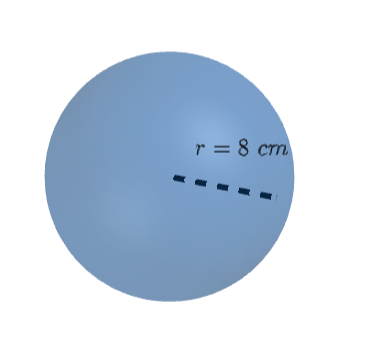Volume of Sphere homework Help at TutorEye

# Best Homework Help For Volume of Sphere

## Top Questions

t schedule 3 people for the day my % scheduled is 78.57% My question is how did the computer come up with the 78.57%?
View More

I would love to get a written solution. I did the other proof tree that you can see in the attaached files. So its supposed to look like that Thank You.
View More

xtbook. i really need in in half an hour
View More

## Volume of Sphere:

The volume of the sphere is 3-dimensional space occupied by it, or by parts of it. It depends upon the radius of the sphere. It is measured under the metric system of units as cubic

Centimeters cm3 , cubic meters m3 , and liter. And, under US standard, the volume is measured as the cubic inch, cubic foot, gallons, pints and fluid ounce.

The following formula is used to find the volume V of the sphere with radius r, and diameter d. And, for hollow spheres, the outer radius is R, and diameter D, with inner radius r, and diameter d.

 Solid Sphere V=4/3πr3 V= π × d3/6 Hollow Sphere V=4/3π (R3 - r3) V=π/6 (D3 - d3)

## Volume of Sphere Sample Questions:

Question 1: Compute the volume of the sphere with radius r=3 cm. Use π=3.14.

Explanation: Plug the value of radius in the volume of sphere formula.

Question 2: Compute the volume of the sphere with diameter d=4 cm. Use π=3.14.

Explanation: Plug the value of diameter in the volume of sphere formula.

Question 3: Compute the volume of the following sphere:Explanation: Plug the value of radius in the volume of sphere formula.

Question 4: Find the amount of air, that can be held by a sphere of diameter d=10 in.

Explanation: Plug the value of diameter in the volume of sphere formula.

Question 5: Calculate the amount of water held by a sphere of radius r=1 m.

Note: 1000 L=1 m3

Explanation: We know the radius of the sphere is r=1 m.

Plug the value of radius in the volume of sphere formula.

Question 6: How many small spherical metal balls with a radius r=2.5 cm, can be made by melting a big metallic sphere of diameter D=10 cm.

Explanation: To solve this problem, we will take the ratio of the volume of the big sphere to the volume of the small sphere.

Question 7: Find the volume of a hollow sphere having outer diameter D=16 in, and an inner diameter of d=9 in.

Explanation: We have given the value of the inner and outer diameter.

Plug the value of diameters in the volume of hollow sphere formula.

Question 8: Calculate the volume of a hollow sphere with an inner radius as r=4 cm, and outer radius as R=6 cm.

Explanation: We have given the value of the inner and outer radius.

Plug the value of radius in the volume of hollow sphere formula.

Question 9: If three spherical wax marbles with radii 5 in, 7 in, and 12 in are mixed to form a big spherical ball. Calculate the diameter of the formed big spherical ball.

Explanation: Now, the volume of the formed spherical ball would equal the collective volume of all three balls.

Question 10: What would be the radius of the sphere having a volume of 904.32 in3. Use π=3.14.

Explanation: We have given the volume, we need to find the radius of the sphere.

V=43r3

Question 1: How to find the volume of a sphere?
Volume of sphere can be calculated by using formula

Where, r is the radius of the sphere.

Question 2: What is the volume of a sphere?

The volume of a sphere is the measurement of space or capacity it occupies. It is a 3-dimensional object with no vertex and no edge. It is round in shape with a fixed radius all around.

Question 3: .How to find the radius of a sphere with the volume?

The radius of a sphere is the distance from its centre to any point on its surface.

We have volume formula as: